# Quiz: Chapter 3 Review Quiz 2 of 12 (1 At one (a) What (b) What th-the...Quiz: Chapter 3 Review Quiz 2 of 12 (1 At one (a) What (b) What th-the that we within 2 5 standard deviations of the mean? Round to the nearest hundredth as needod ) (Round to the nearest handredth as needed) The gasoline prices that are within 2 5 standard deviations of the mean are to (Use ascending order) (c) %isthemiinum percentage of gasoine stations that had prices between SJ 3, and S O Type here to search

We know by Chebyshev's inequality we know that  at least of the distribution's values are within standard deviations of the mean

a) Here k=4, so

So 93.75%

b) Here k=2.5 , so

So 84.00 % prices within 2.5 standard deviation from the mean

As and

c) Since and

So 3.37 to 3.61 prices lies within 2 standard deviations from the mean

Hence the percentage of data is

So 75.00 % data lies within 3.37 to 3.61

##### Add Answer to: Quiz: Chapter 3 Review Quiz 2 of 12 (1 At one (a) What (b) What th-the...
Similar Homework Help Questions
• ### At one point the average price of regular unleaded gasoline was $3.45 per gallon. Assume that...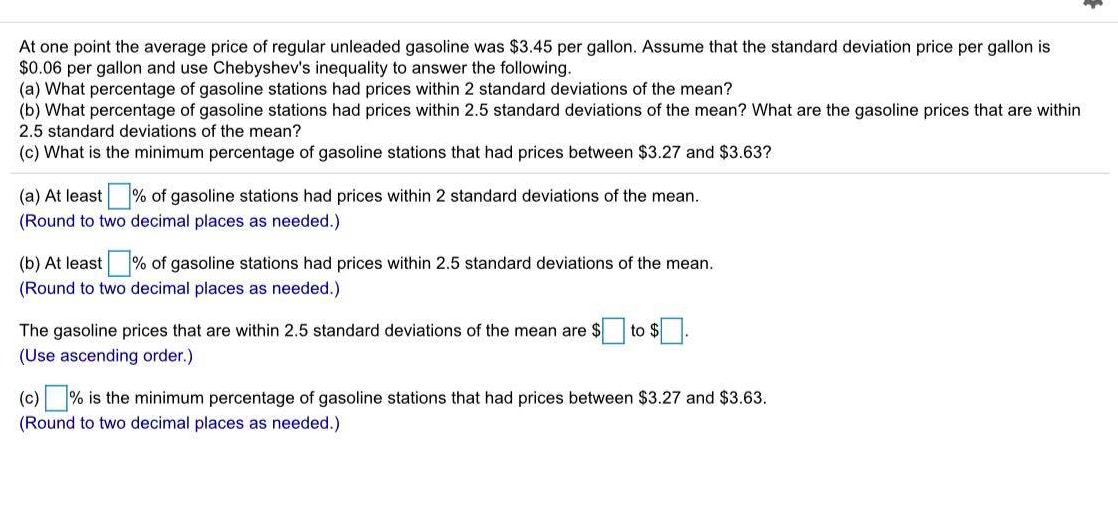At one point the average price of regular unleaded gasoline was$3.45 per gallon. Assume that the standard deviation price per gallon is $0.06 per gallon and use Chebyshev's inequality to answer the following. (a) What percentage of gasoline stations had prices within 2 standard deviations of the mean? (b) What percentage of gasoline stations had prices within 2.5 standard deviations of the mean? What are the gasoline prices that are within 2.5 standard deviations of the mean? (c) What... • ### At one point the average price of regular unleaded gasoline was$3.49 per gallon. Assume that...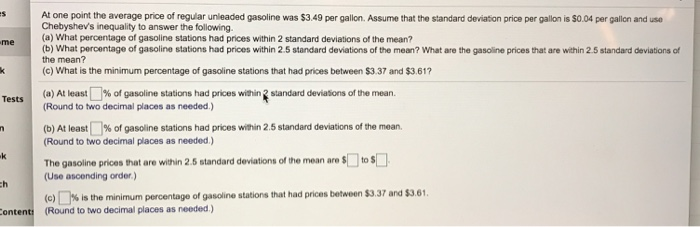At one point the average price of regular unleaded gasoline was $3.49 per gallon. Assume that the standard deviation price per gallon is$0.04 per gallion and use Chebyshevs inequality to answer the following (a) What percentage of gasoline stations had prices within 2 standard deviations of the mean? (b) What percentage of gasoline stations had prices within 2.5 standard deviations of the mean? What are the gasoline prices that are within 2.5 standard deviations of the mean? (c) What...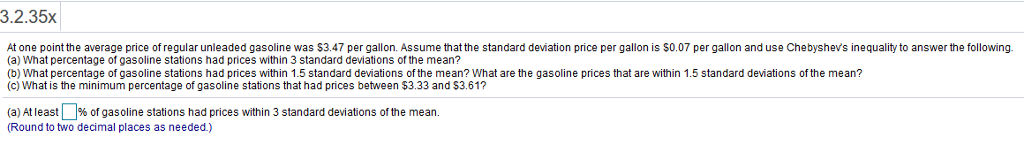3.2.35x At one point the average price of regular unleaded gasoline was $3.47 per gallon. Assume that the standard deviation price per gallon is S0.07 per gallon and use Chebyshev's inequality to answer the following (a) What percentage of gasoline stations had prices within 3 standard deviations of the mean? (b) What percentage of gasoline stations had prices within 1.5 standard deviations of the mean? What are the gasoline prices that are within 1.5 standard deviations of the mean? (c)... • ### 235x Question Help * At one point the average price of regular unleaded gasoline was$3.47...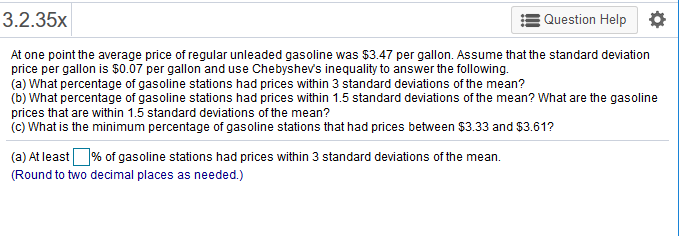235x Question Help * At one point the average price of regular unleaded gasoline was $3.47 per gallon. Assume that the standard deviation price per gallon is$0.07 per gallon and use Chebyshevs inequality to answer thefollowing. (a) What percentage of gasoline stations had prices within 3 standard deviations of the mean? (b) What percentage of gasoline stations had prices within 1.5 standard deviations of the mean? What are the gasoline prices that are within 1.5 standard deviations of the...

• ### Complete parts A through C At one point the average price of regular unleaded gasoline was...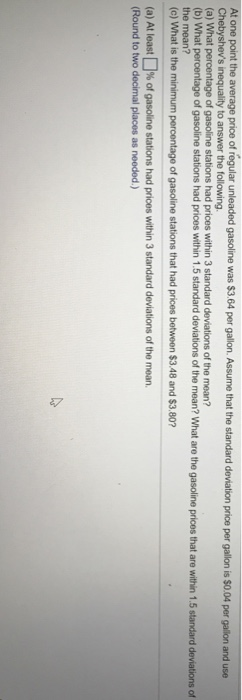Complete parts A through C At one point the average price of regular unleaded gasoline was $3.64 per gallon. Assume that the standard deviation price per gallon is$0.04 per galon and use s inequality to answer the following. (a) What percentage of gasoline stations had prices within 3 standard deviations of the mean? (c) What is the minimum percentage of gasoline stations that had prices between $3.48 and$3.80 (a) At least % of gasoline stations had prices within...

• ### 3.2.35x E Question Help At one point the average price of regular unleaded gasoline was $3.38...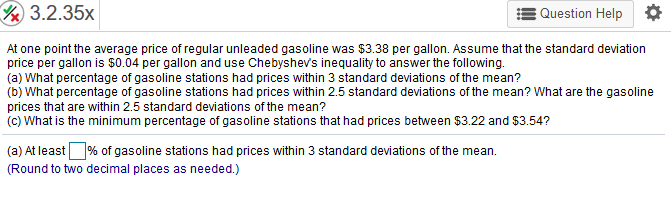3.2.35x E Question Help At one point the average price of regular unleaded gasoline was$3.38 per gallon. Assume that the standard deviation price per gallon is $0.04 per gallon and use Chebyshevs inequality to answer the following. (a) What percentage of gasoline stations had prices within 3 standard deviations of the mean? (b) What percentage of gasoline stations had prices within 2.5 standard deviations of the mean? What are the gasoline prices that are within 2.5 standard deviations of... • ### Show brief calculation for #13 Page S of 8 At one point the average price of...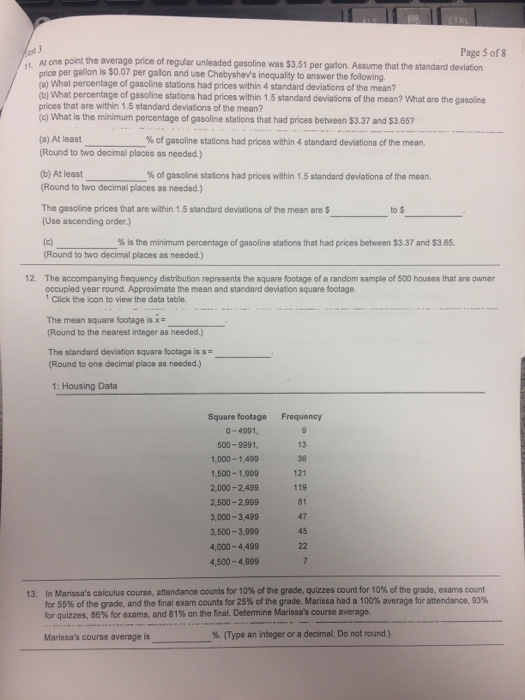Show brief calculation for #13 Page S of 8 At one point the average price of regular unleaded gasoline was$3.51 per gallon. Assume that the standard deviation price per gallon is $0.07 per galon and use Chebyshev's inequality to answer the following a) What percentage of gasoline stations had prices within 4 standard deviations of the mean? (b) What percentage of gasoline stations had prices within 1.5 standard deviations of the mean? What are the gasoline prices that are... • ### At one point the average price of regular unleaded gasoline was$3.49 per gallon. Assume that...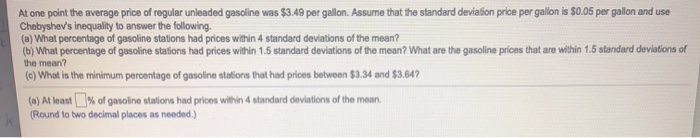At one point the average price of regular unleaded gasoline was $3.49 per gallon. Assume that the standard deviation price per gallon is$0.05 per gallon and use Chebyshev's inequality to answer the following. (a) What percentage of gasoline stations had prices within 4 standard deviations of the mean? (b) What percentage of gasoline stations had prices within 1.5 standard deviations of the mean? What are the gasoline prices that are within 1.5 standard deviations of the mean? (c) What...

Free Homework App